# Best ways to Prepend to a List In Python With ExamplesAuthor - Sanjay

## Introduction

Python is one of the most popular programming languages in the present market , and many developers are using it for various projects. One of the key features of Python is the ability to manipulate lists, and one of the most common operations on lists is to add elements to the beginning of the list. In this article, we will understand the various ways to prepend elements to a list in Python with few examples.

## Pre-Requisistes

A Ubuntu 22.10 server or a Desktop with python 3 installed .

## Basics Of Python Lists

In Python, a list is a collection of items that are ordered and mutable. which means , you can change the contents of the list after it's been created. Lists are denoted by square brackets [] and can contain any type of object, including other lists. Here's an example of a simple list in Python:

``my_list = [1, 2, 3, 4, 5]``

Lists in Python are indexed, meaning that you can access individual items in a list by their position or index within the list. In Python, the first item in a list is always located at index 0.

#### How to Access first item in a List In Python

Here's an example of how to access the first item in the list we just created:

``first_item = my_list``

Since 1 is the first item in the list . Thus , above line would set the variable `first_item` to the value `1`,

Now that we have a very basic understanding of how lists work in Python.

let's move on to how to prepend to a list.

There are variety of ways to prepend a list in python . Lets start with all the examples below .

## 1. Using the insert() method

The `insert()` method is a built-in method in Python that can be used to add an element at a specific position in a list.

To prepend an element to a list, we can use the `insert()` method with an index of 0, which will insert the element at the beginning of the list. Here's an example:

``````linuxt_list = [1, 2, 3]
linuxt_list.insert(0, 0)
print(linuxt_list) # Output: [0, 1, 2, 3]``````

Output:-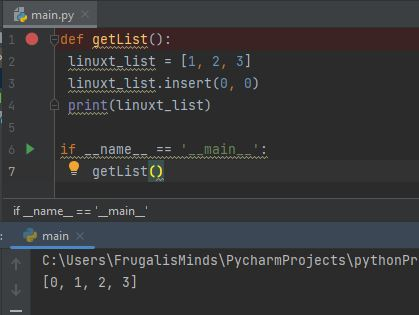## 2 Using the + operator

Next basic way to prepend elements to a list is to use the + operator, which is used for concatenating lists. To prepend an element to a list, we can create a new list with the element we want to prepend, and concatenate it with the original list using the + operator. Here's an example shown below:

``````linuxt_list = [1, 2, 3]
new_element = 
linuxt_list = new_element + linuxt_list
print(linuxt_list) # Output: [0, 1, 2, 3]``````

Output:-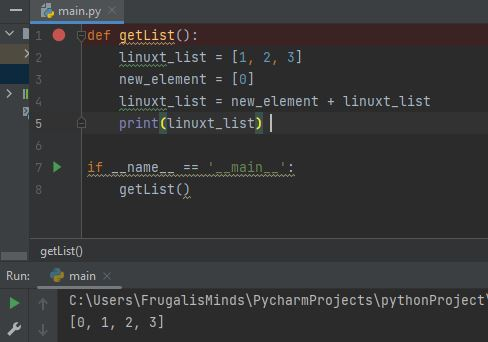## 3. Using the concatenation operator with list

Similar to the + operator, we can also use the list concatenation operator to prepend elements to a list. The list concatenation operator is represented by two consecutive square brackets, and it concatenates two lists.

To prepend an element to a list, we can use the list concatenation operator with a list containing the element we want to prepend. Here's an example shown below:

``````linuxt_list = [1, 2, 3]
new_element = 
linuxt_list = new_element + linuxt_list
print(linuxt_list) # Output: [0, 1, 2, 3]``````

Output:-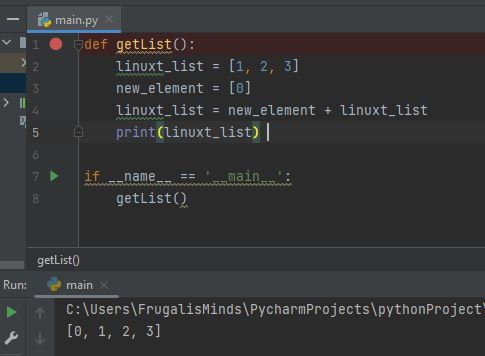## 4. Using the * operator

The * operator is used for repeating a sequence. To prepend an element to a list using the * operator, we can create a new list containing the element we want to prepend, and repeat it once using the * operator. We can then concatenate this new list with the original list using the + operator. Here's an example shown below:

``````linuxt_list = [1, 2, 3]
new_element = 
linuxt_list = new_element * 1 + linuxt_list
print(linuxt_list) # Output: [0, 1, 2, 3]``````

## 5. Using slicing

Slicing is a way to extract a portion of a list. We can also use slicing to prepend elements to a list. To do this, we can slice the original list starting from index 0, and concatenate it with a new list containing the element we want to prepend.

Here's an example shown below:

``````linuxt_list = [1, 2, 3]
new_element = 
linuxt_list = new_element[:] + linuxt_list
print(linuxt_list) # Output: [0, 1, 2, 3]``````

Output:-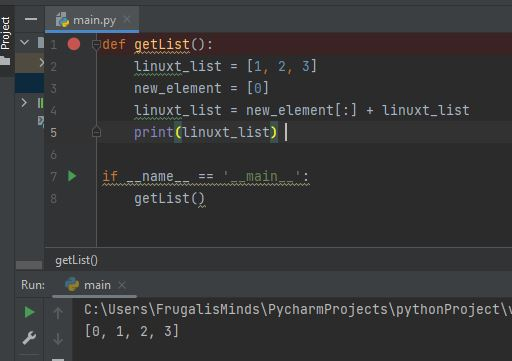## 6.Prepending a List to Another List using Extend

What if we want to prepend an entire list to another list? We can do this using the same method as above, using the `extend()` method. Here's the syntax for using extend() to prepend one list to another: Let's walk through an example of this. Suppose we have the following two lists:

``````linuxt_list = [1, 2, 3]
new_linuxt_list = [4, 5, 6]``````

And we want to prepend new_linuxt_list to linuxt_list. We can do this using the extend() method as follows:

``my_list.extend(new_list)``

After this code executes, linuxt_list will be [4, 5, 6, 1, 2, 3], with new_linuxt_list now at the beginning of the list.

Output:-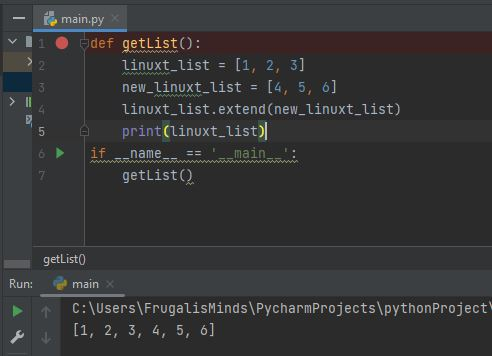## 7.Using the Unpacking Operator

Another way to prepend multiple items to a list is to use the unpacking operator *. The unpacking operator allows us to expand a list into individual items, which we can then pass as separate arguments to a method. Here's the syntax for using the unpacking operator to prepend multiple items to a list:

``linuxt_list = [*new_linuxt_list, *linuxt_list]``

In this code, new_linuxt_list is a list of the items we want to prepend, and linuxt_list is the list we want to prepend them to.

Let's walk through an example to demonstrate this. Suppose we have the following list:

``````linuxt_list = [7, 8, 9]
``````

And we want to prepend the items [4, 5, 6] to the beginning of the list. We can do this using the unpacking operator as follows:

``linuxt_list = [*[4, 5, 6], *linuxt_list]``

After this code executes, linuxt_list will be [4, 5, 6, 7, 8, 9], with the new items now at the beginning of the list.

## Conclusion

Prepending to a list in Python can be accomplished using a few different techniques, including the `insert()` and `extend()` methods, as well as slicing and the unary operator. Which method you choose may depend on the specific requirements of your code, as well as personal preference.

By using these techniques, you can easily add items to the beginning of a list in Python, making it easier to manipulate and work with your data. So the next time you find yourself needing to prepend to a list, remember these techniques and choose the one that best fits your needs.

In summary, you can prepend to a list in Python by using:

• The insert() method
• The extend() method
• Slicing
• The unary + operator

Just keep in mind that each of these techniques has its own advantages and disadvantages, so be sure to choose the one that's best for your specific use case. Happy coding!